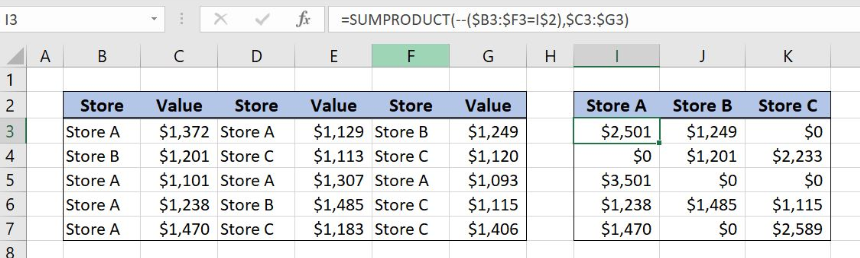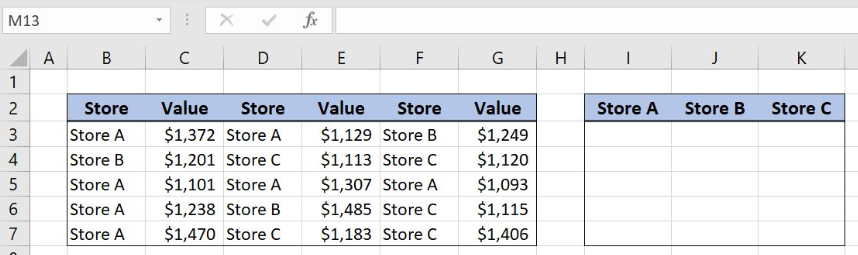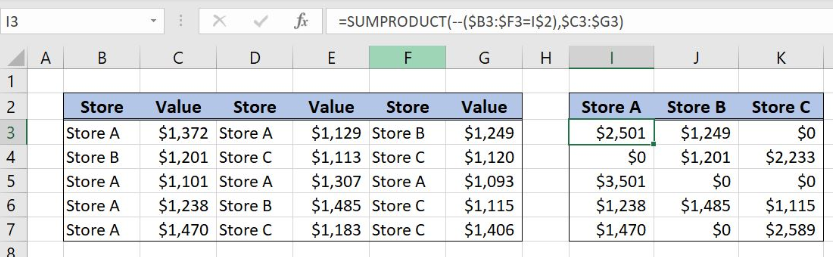Get instant live expert help with Excel or Google Sheets“My Excelchat expert helped me in less than 20 minutes, saving me what would have been 5 hours of work!”

#### Post your problem and you'll get expert help in seconds

Your message must be at least 40 characters
Our professional experts are available now. Your privacy is guaranteed.

# Sum columns based on adjacent criteriaRead time: 25 minutes

With SUMPRODUCT function Excel allows us to sum column values based on the criteria in the adjacent column. This step by step tutorial will assist all levels of Excel users in summing the values from the multiple columns based on the criteria in the neighboring column from the right side.Figure 1. Sum values in each row based on the store

## Syntax of the SUMPRODUCT Formula to Sum With Criteria

`=SUMPRODUCT(--(Criteria_range=Criteria),Sum_range)`

The parameters of the SUMPRODUCT function for counting with criteria are:

• Criteria_range – a cell range where we want to check the criteria
• Criteria – a criteria for summing the cells
• Sum_range – a cell range where we want to sum the data

## Setting up Our Data to Sum Columns Based on Adjacent Criteria

Our first table consists of 6 columns: “Store” (columns B,D,E) and “Value” (columns C,E,G). The second one has three columns: “Store A” (Column I), “Store B” (Column J) and “Store C” (Column K). The idea is to sum the values of Store A, Store B and Store C in each row of the first table and to place the result in the respective column in the second table.Figure 2. Table structure to sum columns based on adjacent criteria

## SUMPRODUCT Function for Summing Values in Each Row Based on the Store

We want to summarize the values for Store A, Store B and Store C for each row of the first table and to place the results in the second table.

The formula looks like:

`=SUMPRODUCT(--(\$B3:\$F3=I\$2),\$C3:\$G3)`

The criteria_range in the SUMPRODUCT function is the cell range B3:F3 while the criteria is the cell I2. The sum_range in the function is the cell range C3:G3.

To apply the SUMPRODUCT function we need to follow these steps:

• Select cell I3 and click on it
• Insert the formula: `=SUMPRODUCT(--(\$B3:\$F3=I\$2),\$C3:\$G3)`
• Press enter
• Drag the formula right to the other cells in the row and then down to the other cells in the column by clicking and dragging the little “+” icon at the bottom-right of the cell.Figure 3. Sum values per store for each row with SUMPRODUCT function

SUMPRODUCT formula part –(\$B3:\$F3=I\$2) is checking if the Store A exist in the array B3:F3. The output of this formula part looks like: `{TRUE;FALSE;TRUE;FALSE;FALSE}`. Double negative sign converts TRUE and FALSE values into 1 and 0: `{1;0;1;0;0}`.

This array is then multiplied with the sum_range array C3:G3: {1372; Store A; 1129; Store B; 1249}. Starting column for the second array is shifted to the right for the one column and the total number of columns in the array is 5 as in the criteria_range array.

As a result, store values are multiplied with the number 1 if the store on the left side is Store A and with 0 if it’s not:

` =SUMPRODUCT({1;0;1;0;0},{1372; Store A; 1129; Store B; 1249})`

SUMPRODUCT function ignores text values in the formula and gives the output array: `{1372,0,1129,0,0,0}`. The final result is number 2501, the sum of the values for the Store A in the first row of the left table.

Most of the time, the problem you will need to solve will be more complex than a simple application of a formula or function. If you want to save hours of research and frustration, try our live Excelchat service! Our Excel Experts are available 24/7 to answer any Excel question you may have. We guarantee a connection within 30 seconds and a customized solution within 20 minutes.

### Did this post not answer your question? Get a solution from connecting with the expert.Another blog reader asked this question today on Excelchat:## Subscribe to Excelchat.coAnother blog reader asked this question today on Excelchat: